Dersleri yüzünden oldukça stresli bir ruh haline sikiş hikayeleri bürünüp özel matematik dersinden önce rahatlayabilmek için amatör pornolar kendisini yatak odasına kapatan genç adam telefonundan porno resimleri açtığı porno filmini keyifle seyir ederek yatağını mobil porno okşar ruh dinlendirici olduğunu iddia ettikleri özel sex resim bir masaj salonunda çalışan genç masör hem sağlık hem de huzur sikiş için gelip masaj yaptıracak olan kadını gördüğünde porn nutku tutulur tüm gün boyu seksi lezbiyenleri sikiş dikizleyerek onları en savunmasız anlarında fotoğraflayan azılı erkek lavaboya geçerek fotoğraflara bakıp koca yarağını keyifle okşamaya başlar

GET THE APP

Dynamic Energy Detection Approach for Spectrum Sensing in Cognitive Radio Networks | OMICS International
International Journal of Advance Innovations, Thoughts & Ideas

# Dynamic Energy Detection Approach for Spectrum Sensing in Cognitive Radio Networks

Pulkit Sharma1,*, Naresh Kumar2

1ME (ECE), Electronics and Communication Engineering Branch, UIET, Panjab University, Chandigarh

2Assistant Professor,Electronics and Communication Engineering Branch, UIET, Panjab University, Chandigarh

*Corresponding Author:
Pulkit Sharma
ME (ECE), Electronics and Communication Engineering Branch
UIET, Panjab University
Chandigarh
E-mail: pulkitsharmay2k@gmail.com

Visit for more related articles at International Journal of Advance Innovations, Thoughts & Ideas

#### Abstract

The current spectrum management scheme is leading to underutilization of spectrum. Cognitive Radio Networks solves the problem of spectrum scarcity by using dynamic spectrum management. Using dynamic spectrum management secondary users can use the licensed spectrum opportunistically. Cognitive Radio Networks can be implemented practically only after detecting those primary bands which are not used by licensed primary user. These free primary bands are technically called spectrum holes and detecting these spectrum holes is called spectrum sensing. New technique for detecting spectrum holes in primary band is proposed in this paper. Various techniques used for spectrum sensing are also described in this paper. Performance of the proposed spectrum sensing method is evaluated under different parameters. Spectrum sensing results for proposed method are compared with the spectrum sensing methods proposed previously. Simulation results shows that the spectrum sensing results for the proposed scheme are better than the previously proposed methods.

#### Keywords

Cognitive Radio Networks, Dynamic Spectrum Management, Spectrum Sensing.

#### Introduction

The paper is arranged in five more sections. In Section II preliminaries and channel model is discussed, section III gives description about Energy Detection. New method called Dynamic Energy Detection is described in Section IV. Simulation results are discussed in Section V and finally the paper is concluded in Section VI.

#### Preliminaries and Channel Model

In order to sense the spectrum whether the channel is free or not we have to consider some channel model or the Here the channel model is taken in accordance with  which states that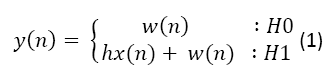(1)

Where

h = Channel Gain.

x(n) = Primary signal transmitted through a wireless channel.

w(n) = Additive Gaussian White Noise is a Gaussian Random Variable with zero mean and Power Spectral Density (PSD) Ɲ0

i.e. w(n) ≈ Ɲ(0, Ɲ0)

Binary Hypothesis:

H0: Signal Absent

H1 : Signal Present

#### Energy Detection

Energy Detection is the most common way of spectrum sensing because of its simplicity and low implementation complexities [3-5]. In an Energy Detector the received signal is first pre filtered by an ideal Band Pass Filter which has Bandwidth ‘W’ and then its output is squared and integrated(summed) over a time interval ‘T’(‘N’) to get the test statistic ‘G’. This test statistic is then compared with the threshold ‘λ’.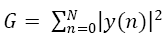(2)

White noise is modelled as a zero mean Gaussian random Variable (GRV) with variance σω2 i.e.

w(n)= Ɲ(0,σω2)      (3)

To make a simple analysis let the signal let the signal can also be modelled as a zero mean Gaussian Random Variable (GRV)

s(n)=σs2      (4)

Due to these assumptions the decision metric M follow a chi – square distribution with 2N degrees of freedom Χ22N and hence can be modelled as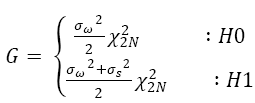(5)

Energy Detector has few challenges

• Selection of threshold for detection primary users.

• Energy Detector has poor performance under low Signal to Noise Ratio (SNR).

• Energy Detector is unable to differentiate interference primary user and noise.

• Energy Detector doesn’t work efficiently for spread spectrum signals.

Two metrics called Probability of Detection (PD)and Probability of False Alarm (PF)are used to calculate the performance of spectrum sensing in Cognitive Radio Networks. Probability of false alarm means the test decides that the primary user is present when it is not present actually while Probability of Detection is detection of an actual primary signal. Both these probabilities are written mathematically in equations (6) and (7) .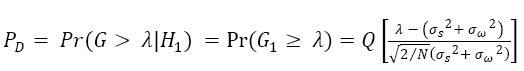(6)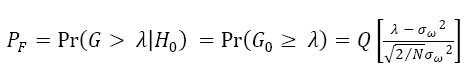(7)

σω2 = Gaussian White Noise Variance.

σs2 = Primary Signal Variance.

N = Number of Samples of received Primary Signal.

λ = Decision Threshold.

Q( ) = Standard Gauss Complementary Accumulated Function.

Choosing the threshold ‘λ’ is very important for the receiver and it is chosen such that a certain amount of False Alarm Rate can be achieved. For a given value of Probability of False Alarm the threshold can be calculated according to relation.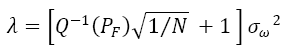(8)

N = Number of Samples of received Primary Signal.

σω2 = Gaussian White Noise Variance.

PF = Probability of False Alarm.

Q-1( ) = Inverse of Standard Gauss Complementary Accumulated Function.

#### Dynamic Energy Detection

Energy detection is described in detail in the previous sections. To increase the effectiveness of Energy Detection a new scheme called Dynamic Energy Detection is proposed in this paper. In this scheme of Energy Detection difference values of square of test statistics are taken. Squared previous value is subtracted from the squared value of current sample. In this technique we get a series of statistics by subtracting squared previous test statistic from squared current statistics according to equation given below.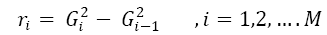(9)

In this technique mean value as the average change of Energy Detection result. The test statistic for this technique is,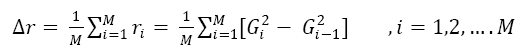(10)

Thus sample of a result are compared with test statistic calculated above and thus the final decision statistic is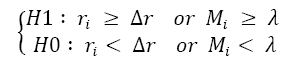(11)

This is the dynamic energy detection scheme proposed for spectrum sensing in Cognitive Radio Networks. Performance of squared energy detection spectrum sensing is calculated using two metrics Probability of Detection (PD) and Probability of False Alarm (PF) and these two metrics are calculated according to the values given in equation (6) and (7).

#### Simulation Results

The proposed system is simulated in MATLAB7 Release 2010a. The simulation is done for the proposed dynamic energy detection. The parameters used to evaluate the performance of proposed method are probability of detection and probability of false alarm. The simulation results are shown in figure 1 shown below.

Here in figure 1 the green ‘*’ line corresponds to a traditional energy detector. The red ‘o’ line corresponds to the differential energy detector proposed in literature. The blue line in this figure shows the performance of the dynamic energy detection proposed in this paper. From the simulation results it is clear that the proposed scheme performs better than the traditional energy detection methods proposed in literature.

#### Conclusion

Energy Detection is described in detail in the starting of this paper. The problem with the traditional Energy Detection is that it cannot differentiate between the noise and primary signal. A novel energy detection approach called squared energy detection is proposed in this paper. The performance of proposed squared energy detection is calculated using performance metrics Probability of detection and Probability of False Alarm. In the proposed method of spectrum sensing the test statistic is compared with the mean value of the difference between the squares of two samples. The chances of detection of signal increase in this method because the test statistic now is compared with the new threshold which is according to the strength of received signal. The simulation results showed that the proposed scheme performs better than the previously proposed schemes in the literature.

--

### Article Usage

• Total views: 14173
• [From(publication date):
July-2012 - Dec 10, 2023]
• Breakdown by view type
• HTML page views : 9660Can't read the image? click here to refresh